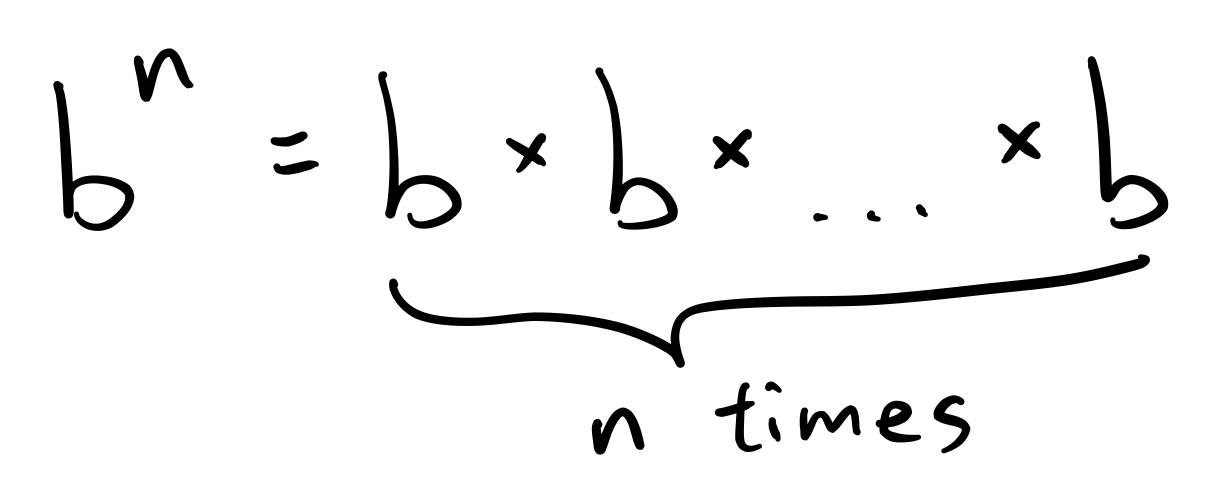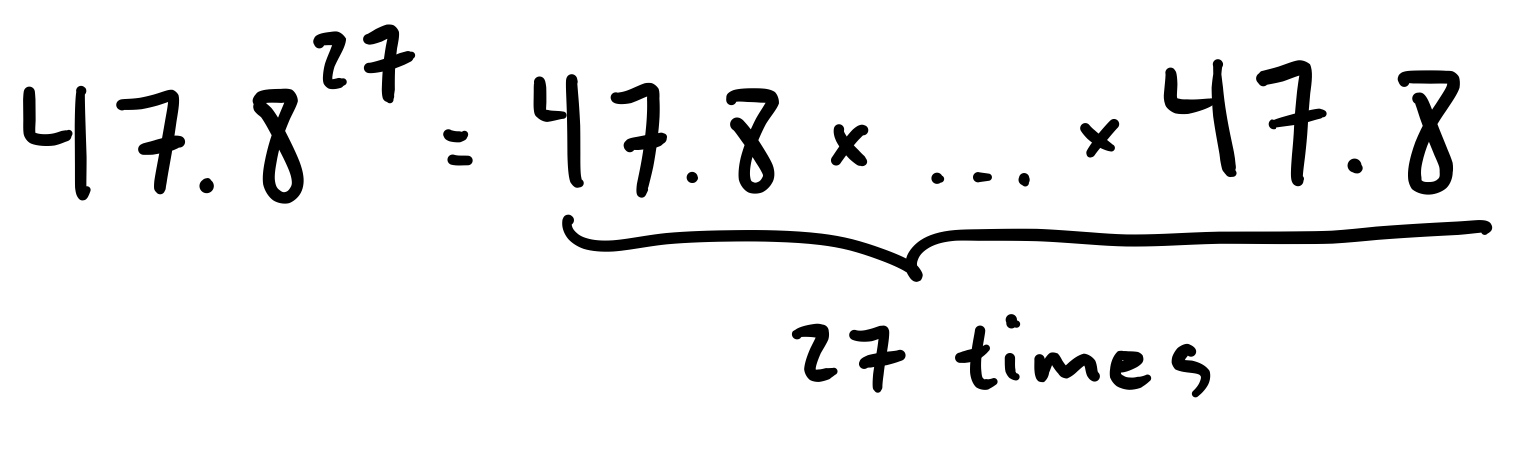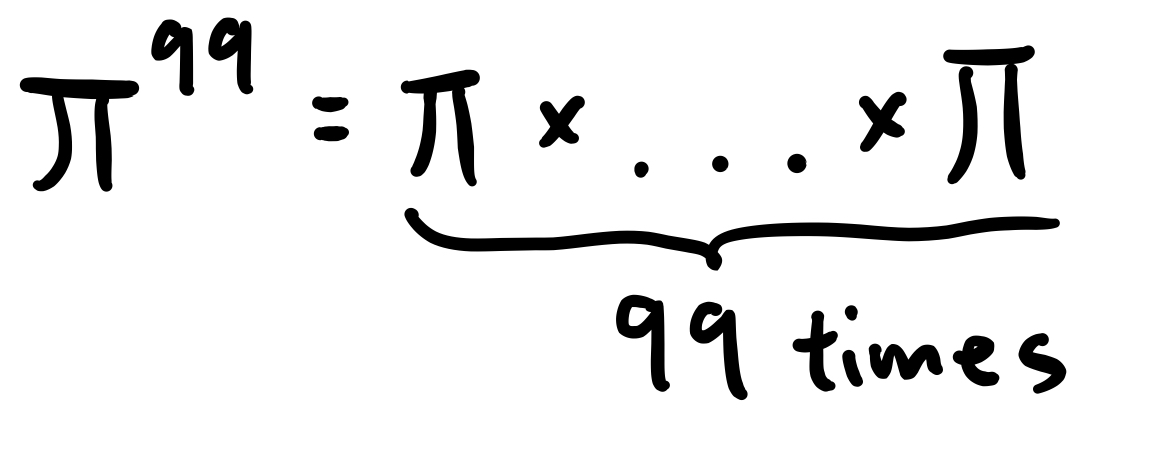Sometimes, we want to multiply a number by itself several times. For example, if we are trying to guess someone’s password, we may want to know how many 5-letter sequences are possible. Following our lessons on combinatorics (see here), we know that there arepossibilities. But we may want an easier way to write this down. The solution is to use exponents. In general,

Rule for Positive ExponentsUsing this rule, we can rewrite our earlier expression as simplyWe call 26 the base and we call 5 the exponent or power. And in general, for,is the base andis the exponent. In English, we say this is “b to the n-th power.” So we would say thatis 26 to the 5th power.

Now, we can take any number and raise it to the power of any positive integer simply by multiplying the number by itself that many times. So, we have:We can even do this for irrational numbers, like:But we can also have negative exponents. When we take a negative exponent, we use the following formula:

Rule for Negative ExponentsIn essence, we flip the base and then take a normal exponent. Here are some examples:Finally, if we raise anything (other than 0) to the 0th power, we get 1. In other words,

Rule for the 0th Power

IfthenFor example:Now, there are some rules for how different things with exponents can be combined.

Multiplication and Division Rules for ExponentsExponent Rule for ExponentsPractice Problems

Simplify the following expressions:

1.2.3.4.5.6.